# Bayesian Approach

 < Day Day Up >

Given a set of forecasts from different methods, we regard the values as sample points from a density. Since at each time we only have a few forecast values, therefore a classical approach for mode estimate seems to be inappropriate. A Bayesian approach is therefore considered. Here we assume that the forecast values do not follow a Gaussian distribution and we assume that it follows the Weibull distribution. This assumption is supported by the empirical evidence. The Weibull distribution is a commonly used distribution in many applications when the density is assumed to be non-Gaussian (see Kotz and Johnson  and the references therein).

We say that a random variable X follows the two-parameter Weibull distribution, denoted as We(a,b), if the density function and the cumulative distribution function are:

 (14)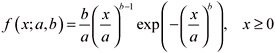(15)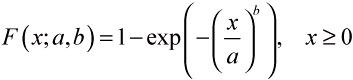The log-likelihood function is given by

 (16)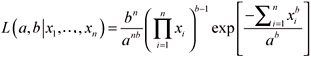The parameter b(b > 0) is a dimensionless shape parameter and a(a > 0) is a scale parameter with the same dimension as X. For a Bayesian approach, we need to choose a joint prior distribution for parameter a and b. As we lack information about this density, we choose the joint prior distribution inversely proportional to the scale parameter a. That is, the joint prior distribution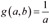. Then the joint posterior is

 (17)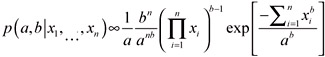It can be shown that the joint posterior distribution and the marginal posterior distribution a and b are proper posterior densities. Therefore important statistics about the parameters such as the posterior mode can be obtained by using the proper joint posterior distribution in equation (17).

 < Day Day Up >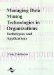Managing Data Mining Technologies in Organizations: Techniques and Applications
ISBN: 1591400570
EAN: 2147483647
Year: 2003
Pages: 174